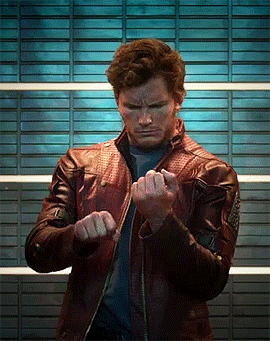---

# 关于GXB 第二次无脑喷我的事^^^^^^^^^^^^^^^^^^^^^^^^^^^^^^^^^^^^^^^^^^^^^^^^^^^^^^^^^^^^^^^^^^^^^^^^^^^^^^^^^^^^^^^^^^^^^^^^^^^^^^^^^^^^^^^^^
GXB你不要无脑喷，你喷的这些ROM是本站访客（他贴吧买的）包括SO核心也是第二天发给我测试的（要不要我给你原始网盘链接？）

BTW1：要和重庆人对骂，我可以说没几个地方的人能有重庆人骂人花样多的... 这种事情小朋友才做，真没意义！
BTW2：不要动不动就主观判断！大家都是灰色的，你之前发IMG不一样盗用老外的资源？好歹我发的IMG不会开机就死全家！对吧！？
BTW3：我的LAKKA更新还要拜你所赐，你不做IMG了，罩不住了，人家拿去用你伤心了！？我说我来做，我来一直更新，我说到了，也一直在更新、在做！要做就要尽量用心做好！
BTW4：所有模拟器IMG镜像更新等7天，P哥等这个人才回应看情况怎么处理，如果不取消单方面的攻击性语言和散布谣言，那我就让这事情变成真的！
^^^^^^^^^^^^^^^^^^^^^^^^^^^^^^^^^^^^^^^^^^^^^^^^^^^^^^^^^^^^^^^^^^^^^^^^^^^^^^^^^^^^^^^^^^^^^^^^^^^^^^^^^^^^^^^^^

So... 那就只有以暴制暴呗，既然说我偷你的东西，那我不偷岂不是对不起你老人家了？